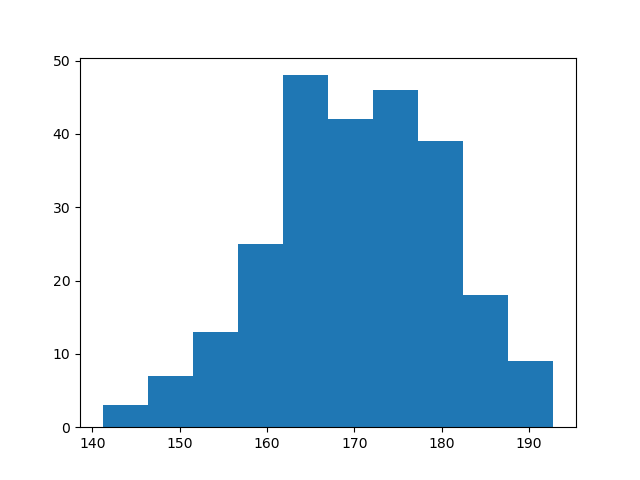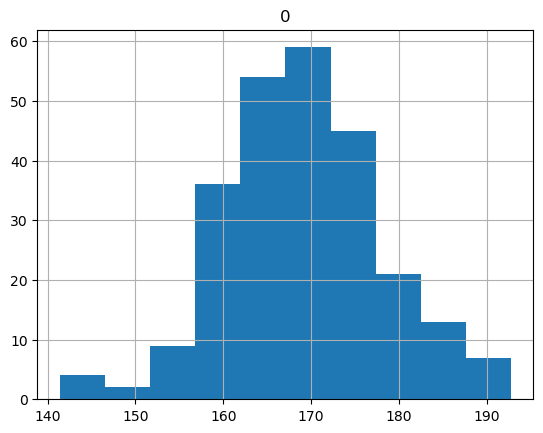# Generating a Histogram with Python: How Would You Do It?

Would you like to know how to generate a histogram in Python? In this tutorial, I will show you how to do it.

The distribution of numerical data can be shown by a histogram (Karl Pearson introduced this term). A histogram is a visual representation of data that uses bars of various heights where each bar divides numbers into ranges. Two modules you can use to plot a histogram in Python are Matplotlib and Pandas.

Let’s find out how to create a histogram!

## What is a Histogram?

Histograms are very important graphs in data analysis. A histogram is a way of displaying the distribution of a numerical set of data using bars of different heights. Taller bars show that more data falls inside that specific range.

We will start by using Python and Matplotlib to plot a histogram. Matplotlib is a library you can use to produce graphs and charts.

## How Can You Generate Data for a Histogram using NumPy?

Before going further, let’s create some dummy data that we will be using to plot histograms using NumPy.

NumPy is a Python library that can handle multi-dimensional arrays.

In order to install NumPy, open the command prompt as an administrator. Then type the following command that will install NumPy on your machine.

The best practice is to execute your applications in a Python virtual environment.

``pip install numpy``

Similarly, you can install Matplotlib using the following Pip command:

``pip install matplotlib``

After importing NumPy, you can generate data by using NumPy arrays. The following code produces random samples from a normal Gaussian distribution.

``````import numpy as np

# Create dummy data points
data = np.random.normal(170, 10, 250)
print(data)``````

The output is:

``````[178.6389057  160.71481129 176.06380975 170.26836416 168.64962801
167.77093268 189.89642816 167.57947841 187.95156914 185.14287433
173.77094473 181.96577219 171.40557555 168.42044648 181.90741839
182.15559495 151.58511408 165.68497833 163.91143081 170.86070342
165.91667438 177.44452444 161.35877875 170.74342034 161.41709815
187.54503422 160.61351112 177.18043424 180.366389   177.56347178
165.48898864 189.19288388 186.5750155  154.66924922 … 170.94541687]``````

You have generated sample data using NumPy.

Now we will move ahead and plot a histogram using this data.

## How Do You Plot a Histogram Using Python and Matplotlib?

We have already generated data using NumPy. We will now use Matplotlib to plot out the first histogram.

The following snippet of code generates a very basic histogram.

``````import matplotlib.pyplot as plt
import numpy as np

data = np.random.normal(170, 10, 250)
plt.hist(data)
plt.show()``````We have successfully plotted our first histogram.

Matplotlib comes with a lot of parameters to customize graphs and charts. We will use them to make the histogram above even better.

In the table below you can see some common parameters:

There are many parameters that are not shown in the table above. You can find them in the official documentation of the matplotlib.pyplot.hist() method.

Now let’s use some of the parameters above to see the difference in the histogram.

``````import matplotlib.pyplot as plt
import numpy as np

data = np.random.normal(170, 10, 250)
plt.hist(data, bins= 20, color='green')
plt.show()``````

The code above is the same with slight changes. We have set the bins to 20 and the color of the histogram to green.

Here is what the histogram looks like:## How To Draw a Histogram Using Pandas?

For the purpose of manipulating and analyzing data, the Python programming language has a software package called Pandas. It allows you to work with time series and mathematical tables.

With the help of Pandas, you can perform data analysis tasks easily and time efficiently.

With Pandas, you can draw histograms using the built-in function hist().

We will generate a histogram using the hist() function based on the data we have already generated.

Have a look at the code below:

``````# import libraries
import numpy as np
import pandas as pd
import matplotlib.pyplot as plt

# generate random data using NumPy
random_data = np.random.normal(170, 10, 250)

# convert the data into a Pandas DataFrame
dataframe = pd.DataFrame(random_data)

# plot histogram using Pandas hist() function
dataframe.hist()``````

In this code, we are generating the same data as we did previously using NumPy, then we are creating a Pandas DataFrame of the generated data.

We are then calling the hist() function on the Pandas DataFrame which produces the following histogram.To show the histogram in Visual Studio code, right-click on the area where your code is and select “Run Current File in Interactive Window“.

You will see the following output:

As an alternative, you can use Jupyter Notebook.

## Conclusion

In this article, we started with the basics of histograms and understood their purpose.

You then implemented Python code to plot histograms of dummy data generated using NumPy. And you have seen how to apply different parameters when generating histograms.

At the end of the article, we have drawn a histogram based on the same dummy data using the Pandas library.

Bonus read: Practice using Pandas. Learn how to calculate the standard deviation of a data set using Pandas.

Related course: Build strong Data Science foundations with “Introduction to Data Science in Python“.